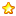# 51单片机控制电机数码管显示转动圈数 c语言代码4 |0 | 2021-4-17 01:30:27 | 显示全部楼层 |阅读模式1. #include <AT89X52.h>       //调用51单片机的头文件

2. //---------------------------------------
3. //数码管字形表，供显示时查询
4. unsigned char code LED=
5. {                          //定义表格一定要使用code，这样会做到程序存储区中
6.     0x3F,                  //"0"的字形表，0B00111111
7.     0x06,                  //"1"的字形表，0B00000110
8.     0x5B,                  //"2"的字形表，0B01011011
9.     0x4F,                  //"3"的字形表，0B01001111
10.     0x66,                  //"4"的字形表，0B01100110
11.     0x6D,                  //"5"的字形表，0B01101101
12.     0x7D,                  //"6"的字形表，0B01111101
13.     0x07,                  //"7"的字形表，0B00000111
14.     0x7F,                  //"8"的字形表，0B01111111
15.     0x6F,                  //"9"的字形表，0B01101111
16. };

17. //---------------------------------------
18. //4位数码管相关I/O设置
19. sbit U165A0=P1^0;           //U165(74HC138)的A0脚接在P1.0口上
20. sbit U165A1=P1^1;           //U165(74HC138)的A1脚接在P1.1口上
21. sbit U165A2=P1^2;           //U165(74HC138)的A2脚接在P1.2口上
22. //---------------------------------------

23. //---------------------------------------
24. //名称: 主函数

25. void main(void)            //主函数,单片机开机后就是从这个函数开始运行
26. {

27.     unsigned int c=0;     //定义一个char型变量，做延时用
28.     unsigned char d=0;     //定义一个char型变量，控制显示位置
29.     unsigned char e=0;     //定义一个char型变量，做延时用
30.     unsigned int  f=0;     //定义一个int型变量，显示内容用，显示内容0-9999
31.         //for(c=0;c<50000;c++)
32.     while(1)               //死循环,单片机初始化后,将一直运行这个死循环
33.     {

34.         for(c=0;c<5;c++);//做一个0-250的循环，不执行其他操作，只为延时
35.         if(++e>200)        //做一个延时，时间到将显示内容加1
36.         {
37.             e=0;
38.                         if(P0_0==1)           //清零，为下一次延时做准备
39.             {if(++f>9999) f=0;}//显示内容加1，因为只有4位显示，超过9999后归零
40.         }
41.         P2=0;              //关一次显示，以免显示出鬼影
42.         if(++d>3) d=0;     //先将d加1，然后判断是否大于3，大于3归零
43.         if(d==0)           //如果d=0,显示千位
44.         {
45.             P1=0x03;       //U165A0=1,U165A1=1,U165A2=0选通数码管的千位进行显示
46.             P2=LED[f/1000];          //将要显示的f的千位提取出来查表后送显示
47.         }
48.         else if(d==1)      //如果d=1,显示百位
49.         {
50.             P1=0x02;       //U165A0=0,U165A1=1,U165A2=0选通数码管的百位进行显示
51.             P2=LED[(f%1000)/100];    //将要显示的f的百位提取出来查表后送显示
52.         }
53.         else if(d==2)      //如果d=2,显示十位
54.         {
55.             P1=0x01;       //U165A0=1,U165A1=0,U165A2=0选通数码管的十位进行显示
56.             P2=LED[(f%100)/10];      //将要显示的f的十位提取出来查表后送显示
57.         }
58.         else               //如果d=3,显示个位
59.         {
60.             P1=0x00;       //U165A0=0,U165A1=0,U165A2=0选通数码管的个位进行显示
61.             P2=LED[f%10];            //将要显示的f的个位提取出来查表后送显示
62.         }
63.     }
64. }

 本版积分规则 回帖后跳转到最后一页302主题 124积分E币
124